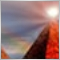Heikin Ashi (better formula)467

Can someone do this program?

From an article made by BNP-Paribas it seems that we can have better representation with Heikin Ashi if we made the following modified Heikin-Ashi candlesticks as follows:

a. haOpen, haHigh and haLow according to Dan Valcuformulas.

b. haClose is calculated first according to the formula (Open+Close)/2+(((Close-Open)/(High-Low))*ABS((Close-Open)/2)), then smoothed with a 2 days (bars) trader adaptive moving average (could be a 3-period T3 moving average or a 2-period Kaufman moving average).

haClose = (O+H+L+C)/4

haOpen = (haOpen (previous bar) + haClose (previous bar))/2

haHigh = Maximum(H, haOpen, haClose)

haLow = Minimum(L, haOpen, haClose)467

{_T3Average

By Bob Fulks, modified by Alex Matulich 4/2003

The T3 Average is essentially a low-pass filter, as are the

traditional moving average and exponential moving average. The T3

Average, however, exhibits a steeper rolloff, resulting in better

filtering of high-frequency noise while better preserving the

low-frequency components of a time series.

This function is an EasyLanguage version of the algorithm described

in the January 1998 issue of TASC, p57, "Smoothing Techniques for

More Accurate Signals" by Tim Tillson. It is translated from

the MetaStock code presented in the article. The function allows

a variable length as input.

The variable b (also called "Hot") is a damping coefficient. The

suggested value of b is 0.7, but this value slightly amplifies

low-frequency components. b=0.5 seems better for having a flat

response at low frequencies. A smaller value of b will result in

too much attenuation of low frequencies.

The Length parameter is divided by two to make the T3 Average's lag

equivalent to the lag of the traditional moving averages. This way

you can use the T3 Average as a drop-in replacement for Average or

xAverage, and get the same lag but better noise filtering.

The variable "b" is substituted for the variable "a" used in the

article since "a" is a reserved word.

}

Inputs: Price(NumericSeries), Length(NumericSimple);

Variables: b(0.5), b2(b*b), b3(b2*b),

e1(Price), e2(Price), e3(Price), e4(Price), e5(Price), e6(Price),

c1(-b3), c2(3*(b2+b3)), c3(-3*(2*b2+b+b3)), c4(1+3*b+b3+3*b2),

N(0), w1(0), w2(0);

N = Length;

if N < 1 then N = 1;

N = 1 + 0.5*(N-1); {makes lag equivalent to Moving Average}

w1 = 2 / (N + 1); w2 = 1 - w1;

e1 = w1*Price + w2*e1;

e2 = w1*e1 + w2*e2;

e3 = w1*e2 + w2*e3;

e4 = w1*e3 + w2*e4;

e5 = w1*e4 + w2*e5;

e6 = w1*e5 + w2*e6;

_T3Average = c1*e6 + c2*e5 + c3*e4 + c4*e3;318

T3

Try this one...

Raff

bunder:
{_T3Average

By Bob Fulks, modified by Alex Matulich 4/2003

The T3 Average is essentially a low-pass filter, as are the

traditional moving average and exponential moving average. The T3

Average, however, exhibits a steeper rolloff, resulting in better

filtering of high-frequency noise while better preserving the

low-frequency components of a time series.

This function is an EasyLanguage version of the algorithm described

in the January 1998 issue of TASC, p57, "Smoothing Techniques for

More Accurate Signals" by Tim Tillson. It is translated from

the MetaStock code presented in the article. The function allows

a variable length as input.

The variable b (also called "Hot") is a damping coefficient. The

suggested value of b is 0.7, but this value slightly amplifies

low-frequency components. b=0.5 seems better for having a flat

response at low frequencies. A smaller value of b will result in

too much attenuation of low frequencies.

The Length parameter is divided by two to make the T3 Average's lag

equivalent to the lag of the traditional moving averages. This way

you can use the T3 Average as a drop-in replacement for Average or

xAverage, and get the same lag but better noise filtering.

The variable "b" is substituted for the variable "a" used in the

article since "a" is a reserved word.

}

Inputs: Price(NumericSeries), Length(NumericSimple);

Variables: b(0.5), b2(b*b), b3(b2*b),

e1(Price), e2(Price), e3(Price), e4(Price), e5(Price), e6(Price),

c1(-b3), c2(3*(b2+b3)), c3(-3*(2*b2+b+b3)), c4(1+3*b+b3+3*b2),

N(0), w1(0), w2(0);

N = Length;

if N < 1 then N = 1;

N = 1 + 0.5*(N-1); {makes lag equivalent to Moving Average}

w1 = 2 / (N + 1); w2 = 1 - w1;

e1 = w1*Price + w2*e1;

e2 = w1*e1 + w2*e2;

e3 = w1*e2 + w2*e3;

e4 = w1*e3 + w2*e4;

e5 = w1*e4 + w2*e5;

e6 = w1*e5 + w2*e6;

_T3Average = c1*e6 + c2*e5 + c3*e4 + c4*e3;
Files:202

best indicator i have ever seen

great indicator very nice work

it wotrks even on trhe 1 minute

can we make a signal when the color turn from red to blue and vice vers

pls see the attached chart of gbp of today

great work guys202

gbp chart 1 min signa needed

gbp chart 1 min signa needed

Files:
gbp_1min.jpg 41 kb318

See post #3...

Raff

crazybunny:
great indicator very nice work

it wotrks even on trhe 1 minute

can we make a signal when the color turn from red to blue and vice vers

pls see the attached chart of gbp of today

great work guys467

Thanks Raff!

Wonderful work again!1187

Hi Raff and everyone - is it possible to make a simple expert based on the arrows ? Thanks a lot !318

I think there is an EA based on HAMa somewhere at forum...

Raff

leeb:
Hi Raff and everyone - is it possible to make a simple expert based on the arrows ? Thanks a lot !7

sorry guys i hate to sound stupid but could anyone please explain in simple terms what the indicator is doing. Also Bunder, do you mind giving us a link to the BNP Paribas article. Thanks.

Wahomeron52

Thanks, Raff

Great Work, Raff. Thank you so much.

Bunder, thank you for posting it here.

Cheers

- Sukhen